# 直线的方程

y = mx + b

（或在英国 "y = mx + c"看下面

### 这代表什么？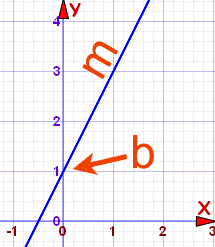陡度 （或坡度、或斜率) Y 截距

y = 向上有多高

x = 向右有多远

m = 陡度（坡度）或斜率（线有多陡）

b = Y截距（线与y轴的相交点）

### 怎样去找"m"和"b"？

• b很容易：看看线在哪里与Y轴相交。
• m（斜率） 则需要一些计算：
 m =  Y 的改变 X 的改变## 例子 1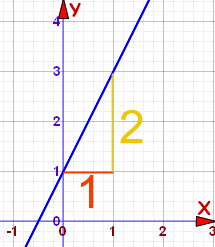m = 2 1 = 2

b = 1 （线与 Y轴的相交点）

 因此： y = 2x + 1

……选 x的任何值，解出 y

y = 2×1 + 1 = 3

y = 2×7 + 1 = 15

## 例子 2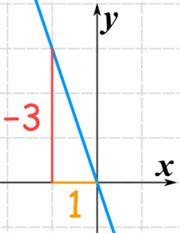m = −3 1 = −3

b = 0

 所以：y = −3x

## 例子 3：垂直线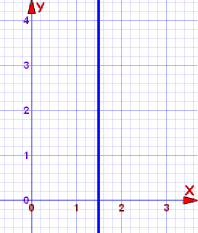x = 1.5

## 上升和奔跑

 有时，在英语使用 "rise（上升）" 和 "run（奔跑）"这两个字。 Rise（上升）是向上有多高 Run（奔跑）是向右有多远 所以斜率 "m"是： m = rise（上升） run（奔跑） 可能这样比较容易记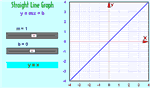## 附注：

### 国家 注意：

 在美国、澳大利亚、加拿大、厄立特里亚、伊朗、墨西哥、葡萄牙、菲律宾、沙特阿拉伯是这样表示： y = mx + b 在英国、澳大利亚（也有）、巴哈马、孟加拉国、比利时、文莱、保加利亚、塞浦路斯、埃及、德国、加纳、印度、印度尼西亚、爱尔兰、牙买加、肯尼亚、科威特、马来西亚、马拉维、马耳他、尼泊尔、阿曼、巴基斯坦、秘鲁、波兰、新加坡、所罗门群岛、南非、斯里兰卡、土耳其、阿拉伯联合酋长国、赞比亚和津巴布韦 y = mx + c 在阿富汗、阿尔巴尼亚、阿尔及利亚、巴西、中国、捷克共和国、丹麦、埃塞俄比亚、法国、黎巴嫩、荷兰、科索沃、吉尔吉斯斯坦、挪威、罗马尼亚、 韩国、西班牙，突尼斯和越南： y = ax + b 在阿塞拜疆、中国、芬兰、俄罗斯和乌克兰： y = kx + b 在希腊： ψ = αχ + β 在意大利： y = mx + q 在日本： y = mx + d 在古巴和以色列： y = mx + n 在罗马利亚： y = gA + C 在拉脱维亚和瑞典： y = kx + m 在塞尔维亚和斯洛文尼亚： y = kx + n 在你的国家： 告诉我们！

……意思都一样，只是用了不同的字母。